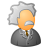# QlikView App Dev

Discussion Board for collaboration related to QlikView App Development.

Announcements
May 18th 10AM ET, Live Chat, bring your QlikView questions. REGISTER
cancel
Showing results for
Did you mean:Partner

## Set Analysis with If()

Dear All,

I have a problem with a Set Analysis. Actually, a want in some cases to get the results of one Set Analysis ELSE get the result of another. Cause is a little bit more complicated (at least in my head right now!), I attached a sample. I want to get the results in pivot table.

Helen

8 RepliesMaster II

Eleni,

This approach won't work unfortunately. What you should keep in mind about set analysis is that it is calculated once per chart. For that reason, you can't use it to check which dimension you are currently in--only a conditional function like IF can do that. However, if I understand your question, this syntax should do the same thing more elegantly:

if(match(CostElement,'R1','R2','R3','R4'),

sum({\$<RM1_Year={\$(=Only(RM1_Year))}, RM1_Period={"<=\$(=Only(RM1_Period))"}>} RM1_Period_Value),

sum({\$<RM1_Year={\$(=Only(RM1_Year))}, RM1_Period={\$(=Only(RM1_Period))}>} RM1_Period_Value)

)

Regards,Partner
Author

First of all I want to thank you for your quick response. Your suggestion it would be the answer to my problem only if in the pivot table we get the correct number to the total (which is the most important for me).

Am I doing something wrong? Do you have to suggest anything else?

Thanks a lot,

HelenMaster II

I think the incorrect total is being caused by a problem with your data model--you have a synthetic key table in there. Try to get rid of that so your CostData and ProdKilos tables are linked to each other by 1 key only.Partner
Author

I'm gonna try this and i will inform you.

Regards,

HelenPartner
Author

although I have avoid the Synthetic Key and the Data Model is ok, the total of this expression in the pivot table display false results (the total in the Straight table is correct). Practicaly, it seems to do Expression Total instead of sum of Rows although in Total Mode the sum of Rows is marked.

Thanks,

HelenMaster II

Helen,

If you want sum of rows in a pivot table to match your current logic for the straight table, you would need to replace the expression with something like this:

sum(aggr(
if(match(CostElement,'R1','R2','R3','R4'),

sum({\$<RM1_Year={\$(=Only(RM1_Year))}, RM1_Period={"<=\$(=Only(RM1_Period))"}>} RM1_Period_Value),

sum({\$<RM1_Year={\$(=Only(RM1_Year))}, RM1_Period={\$(=Only(RM1_Period))}>} RM1_Period_Value)

)
,Desc))

But make sure this is actually what you want and it's not double-counting.

Regards,Partner
Author

I want to thanks you one more time for your response.

I have already tried this but if you put it in the chart you will see that although the total are right, all the other values (cell values) are wrong.

Regards,

HelenMaster II

Helen,

I still think there's an underlying problem with the data model--the "BusinessUnit_Description" and "Desc" are not linked properly between themselves. You can try this expression, but the total is slightly different:

sum(aggr(
if(match(CostElement,'R1','R2','R3','R4'),

sum({\$<RM1_Year={\$(=Only(RM1_Year))}, RM1_Period={"<=\$(=Only(RM1_Period))"}>} RM1_Period_Value),

sum({\$<RM1_Year={\$(=Only(RM1_Year))}, RM1_Period={\$(=Only(RM1_Period))}>} RM1_Period_Value)

)

Alternatively, you can "trick" QlikView into showing you one expression for the total row and a different one for the cell rows:

if(Dimensionality()<>0,

sum(aggr(

if(match(CostElement,'R1','R2','R3','R4'),

sum({\$<RM1_Year={\$(=Only(RM1_Year))}, RM1_Period={"<=\$(=Only(RM1_Period))"}>} RM1_Period_Value),

sum({\$<RM1_Year={\$(=Only(RM1_Year))}, RM1_Period={\$(=Only(RM1_Period))}>} RM1_Period_Value)

)

sum(aggr(

if(match(CostElement,'R1','R2','R3','R4'),

sum({\$<RM1_Year={\$(=Only(RM1_Year))}, RM1_Period={"<=\$(=Only(RM1_Period))"}>} RM1_Period_Value),

sum({\$<RM1_Year={\$(=Only(RM1_Year))}, RM1_Period={\$(=Only(RM1_Period))}>} RM1_Period_Value)

)

,Desc))

)

However, once you start writing expressions like this, the application quickly spins out of control. I suggest you start by investigating why showing BusinessUnit_Description and Desc in the same chart leads to different results that just using Desc as a dimension. My guess would be that it has something to do with the density of BusinessUnit_ID (that it's populated 72% of the time while CostElement is populated 67%).

Regards,